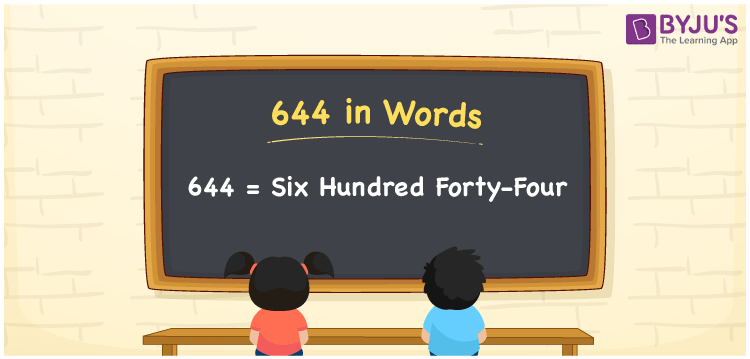# 644 in Words

644 in words is written as Six hundred forty-four. In both the International System of Numerals and the Indian System of Numerals, 644 is written as Six hundred forty-four. The number 644 is a Cardinal Number as it represents some quantity. For example, the “cost of the book is rupees 644”.

 644 in Words Six hundred forty-four Six hundred forty-four in Number 644

## 644 in English Words

We write 644 in English Words using the letters of the English alphabet. Therefore, we read 644 in English as “Six hundred forty-four”.## How to Write 644 in Words?

To write 644 in words, we shall use the place value chart. In the place value chart, write 6 in the hundreds, 4 in the tens, and 4 in the ones, respectively. Now let us make a place value chart to write the number 644 in words.

 Hundreds Tens Ones 6 4 4

Thus, we can write the expanded form as

6 × Hundred + 4 × Ten + 4 × One

= 6 × 100 + 4 × 10 + 4 × 1

= 600 + 40 + 4

= 644

= Six hundred forty-four.

644 is a natural number, the successor of 643 and the predecessor of 645.

644 in words – Six hundred forty-four

• Is 644 an odd number? – No
• Is 644 an even number? – Yes
• Is 644 a perfect square number? – No
• Is 644 a perfect cube number? – No
• Is 644 a prime number? – No
• Is 644 a composite number? – Yes

## Frequently Asked Questions on 644 in Words

Q1

### How to write 644 in words?

644 in words is written as Six hundred forty-four.
Q2

### How to write 644 in the International and Indian System of Numerals?

In both, the system of numerals, 644 in words, is written as Six hundred forty-four.
Q3

### What is the successor of 644?

The successor of 644 is 645.